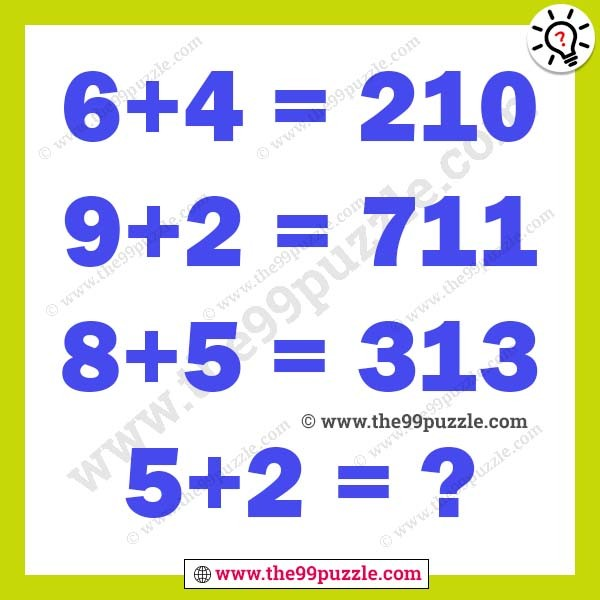# Tricky maths logical puzzle question – Puzz155

This tricky maths logical puzzle question can challenge your mind. Test your mathematical as well as logical reasoning skills with the brain teaser. In this tricky maths logical puzzle question, there are some logical equations. Can you solve these logical equations?

6+4=210

9+2=711

8+5=313

5+2=?###### Explanation:

6+4=(6−4)(6+4)=210

9+2=(9−2)(9+2)=711

8+5=(8−5)(8+5)=313

5+2=(5−2)(5+2)=37

1.